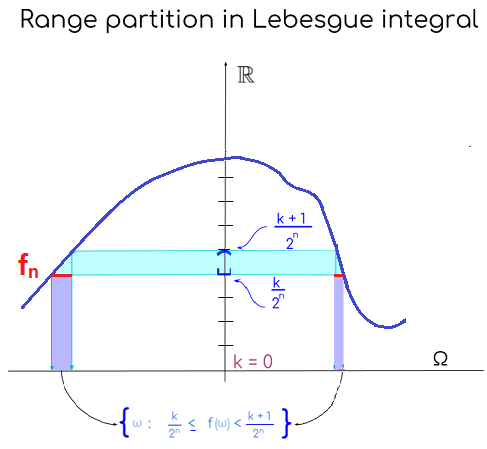### ABSTRACT INTEGRATION:

Abstract integration relies on a measurable space, $$(\Omega,\mathcal F, \mu)$$ and an $$\mathcal F$$-measurable function, $$f: \Omega \to \mathbb R,$$ which in probability theory is a random variable.

The integral

$\int_A f \;\mathrm d\mu$

is the integral over a measurable set $$A$$ of an $$\mathcal F$$-measurable function, $$f$$ with respect to a measure $$\mu.$$

In the specific case when $$(\Omega,\mathcal F, \mu)= (\mathbb R,\mathcal B(\mathbb R), \lambda),$$ i.e. $$f: \mathbb R \to \mathbb R$$ is a Borel-measurable function with the Lebesgue measure $$\lambda,$$ then $$\int f\;\mathrm d \lambda$$ is called the Lebesgue integral. Another specific case is the expectation of a random variable $$X:\Omega \to \mathbb R$$ on a probability space is defined as $$\int X \; \mathrm d\mathbb P.$$

If the integral occurs over the entire sample space it will be implicit in the notation:

$\int_\Omega f \;\mathrm d\mu= \int f \;\mathrm d\mu$

Simple functions assume finitely many values in their image, and can be written as

$f(\omega)= \sum_{i=1}^n a_i \mathbb I_{A_i}(\omega), \quad \forall \omega \in \Omega$

where $$a_i \geq 0, \forall i \in \{1,2,3, \dots, n\},$$ and $$A_i \in \mathcal F, \forall i.$$

Given the finite values in the image of the simple function $$f,$$ corresponding to each of $$n$$ disjoint subsets of $$\Omega,$$ such that $$\bigcup_{i}A_i=\Omega,$$ a simple function can indeed be written as:

$f(\omega)=a_1 \mathbb I_{A_i}(\omega) + a_2 \mathbb I_{A_2}(\omega)+\cdots +a_n\mathbb I_{A_n}(\omega) =\sum_{i=1}^n a_i \mathbb I_{A_i}(\omega).$

indicating that $$a_i$$ will the only value in the sum if it matches the subset $$A_i$$ according to the $$i$$ indexation, akin to a for-loop call in programming: only the $$a_i$$ corresponding to $$\mathbb I_{A_i} (\omega)=1,$$ i.e. when the outcome is in the measurable set $$A_i,$$ is ultimately represented.

The definition of the abstract integral of non-negative functions $$f: \Omega \to \mathbb R_+$$ is defined as the supremum of collection of simple functions that approximate $$f$$ from below:

Given a collection of simple functions $$q: \Omega \to \mathbb R_+$$ denoted $$S(f)=\{s_1, s_2, \dots, s_N\},$$ for some $$N \in \mathbb N,$$ such that for all $$\omega \in \Omega$$ it fulfills $$q(\omega)\leq f(\omega),$$ the abstract integral is defined as

$\int_\Omega f \;\mathrm d\mu=\sup_{q \in S(f)} \int q \;\mathrm d\mu=\sup\left(\sum_{q\in S(f)} q \; \mu (\text{preim}\left(\{q\})\right) \right)$

There is still need to a practical method to approximating or computing this integral. The following is such a method:

$\int_\Omega f \;\mathrm d\mu = \lim_{n \to \infty} \int f_n \mathrm d\mu$ where

$f_n(x) = \sum_{k=0}^{n2^n-1}\frac{k}{2^n}\; \chi_{\frac{k}{2^n}\leq f(x)<\frac{k+1}{2^n}}\; +\; n\,\chi_{f(x) \geq n}$

and letting $$n \to \infty.$$

The range or codomain of the function $$f$$ is partitioned fixing a maximum value $$n$$ from $$k=0$$ to $$k = n$$ at intervals $$\frac{k}{2^n}$$ in order to define a sequence of simple functions $$f_n$$ with value $$n$$ if $$f(\omega)$$ is equal or greater than $$n,$$ and otherwise with the lowest value for that interval, $$n-\frac{1}{2^n}.$$Coding a concept helps, so I tried doing so for this post, illustrating the Lebesgue integral of $$y = x^2$$ between $$[0,1]$$. The code is here, and the output looks like this: